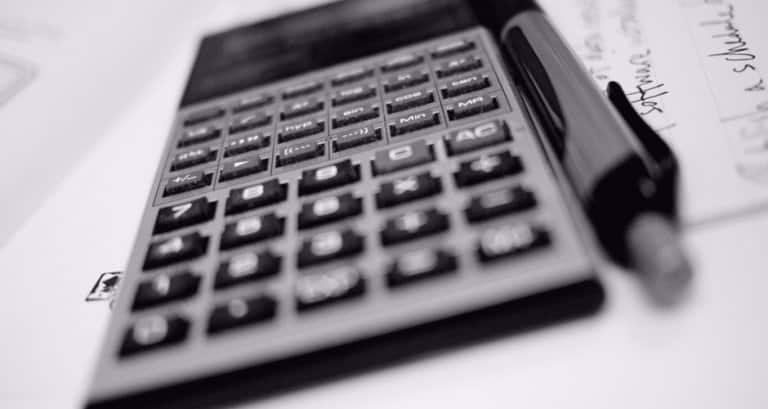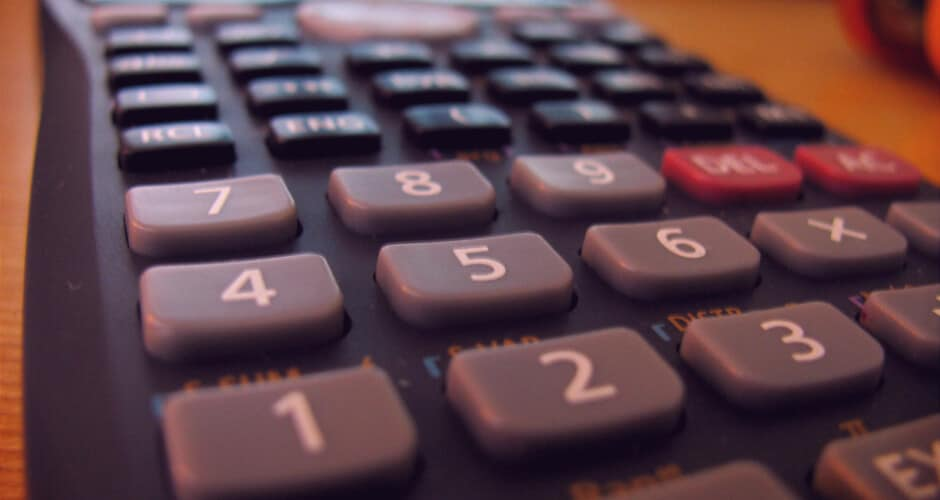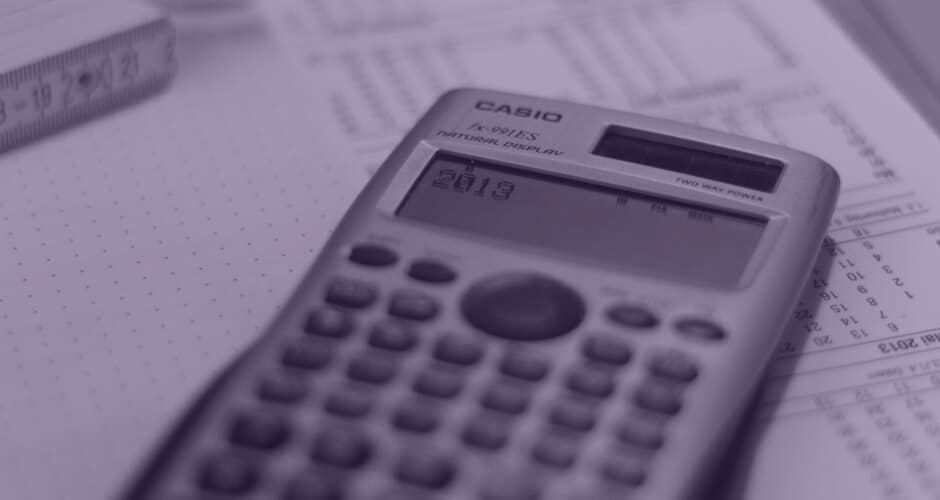Updated on: February 6, 2018

# The Best Scientific Calculator: Buyer’s Guide with Ranking & Reviews

A one-stop resource for finding the scientific calculator you need.I’m a self-proclaimed “math nerd,” and I’m so excited for this opportunity to talk about scientific calculators – what they are, when to use one, the best models, figuring out which model is right for you, and the differences between calculator types.

First, are you sure that you need a scientific calculator and not a graphing calculator? This article will explain the differences in several spots, so if you're confused keep reading.

## Finding the Best Scientific Calculator for Your Needs

Why do you want a scientific calculator? Scientific calculators are much cheaper than graphing calculators, but almost every student will graduate onto a graphing calculator before graduating high school.

If you are a student, the best scientific calculator for you will likely depend on your intended academic (or career) path. If you’re searching for the best scientific calculator because you need specific functionality and want the best deal, you’ll want to skip to the calculator rankings.

Related: Free Wolfram Alpha Alternative that Shows all the Steps

### Types of Calculators on the Market

For all intents and purposes, there are three kinds of calculators on the market:

• Four function ( +, -, x, ÷,=)
• Scientific (sin, cos, tan, ln, log, exp, π , 1/x, nPr, nCr, b/c, a b/c) ⇐ We’re focusing on this type
• Graphing (statistics, matrices, graphing)

I’ll discuss more about incorporating a calculator into childhood education later in the guide, but for now here are the usual grade appropriateness “standards.”

• Four function Elementary school
• Scientific calculators Algebra I, Algebra II, Geometry, Math Analysis, and Geometry Concepts
• Graphing calculators Algebra II, Trigonometry, A.P. Statistics, and everything Calculus

Graphing calculators become almost mandatory for most 9th grade students.

### Calculators Allowed on the SAT

This is a common concern when buying a school calculator, but there is very little to worry about. During the math portion of the SAT/PSAT, students can use any calculator with two exceptions. The calculator cannot have 1) a QWERTY keyboard or 2) paper tape.

### Calculators for A.P. Calculus & A.P. Statistics Courses & Tests

Standardized A.P. Calculus and A.P. Statistics courses in the United States heavily incorporate graphing calculators into all aspects of curriculum. Standard calculator recommendations are as follows:

• A.P. Statistics recommended calculator: TI-83 or TI-84
• A.P. Calculus recommended calculator: TI-83, TI-84, or TI-86
• Banned calculators: TI-89 and TI-92

## Rankings: The Best Scientific Calculator

There is additional informational material below this section that should answer any additional questions you might have.

All calculators listed here are approved for use on the SAT, ACT, and AP exams.

### The Best Mid-Range Scientific Calculator

When comparing the Texas Instruments scientific calculators, there are two that stand out. The TI-30XS MultiView and the TI-36X Pro. The TI-30XS MultiView stands out because it has every feature that the lower models have (TI-30Xz, TI-30X IIS, and TI-34 MultiView) plus more and is only a few bucks more.

This is a great "transition" calculator to be used before graduating to the TI-84 graphing calculator.

Grade/class appropriateness: General Math, Pre-Algebra, Algebra 1 & 2, General Science, Geometry, Statistics, Biology, and Chemistry.

Not appropriate for: Pre-Calculus, Calculus, Physics, Computer Science, or Engineering.

Key features: Display has four-lines, 1 & 2 variable statistics, fraction/decimal conversion, step-by-step fraction simplification, edit/cut/paste entries, and is solar + battery powered.

### The Best Non-Graphing Calculator for Engineering, Chemistry, & Computer Science

This is hands down the best non-graphing TI calculator on the market. When I compare this calculator with graphing calculators like the TI-84, the TI-36X Pro can do everything except the graphing portion.

This is a big jump in functionality! Now we’re talking about some serious computing. When you compare the features-to-price-ratio on all TI machines, this is the most "bang for your buck" calculator of anything market. Additionally, this calculator shines because it incorporates statistical analysis and Boolean logic operations.

This calculator’s main “non-graphing competitor” is the TI-30X. There is no comparison, the TI-36X is a far superior calculator. It is hard to explain without having both models in your hands, but the button layout and organization is much better on the 36X. Frequently used functions like exponentials have their own buttons, while lower models like the 30X force you to click “2nd” and then another one+ button(s). Furthermore, all of the trig and inverse trig functions are located conveniently.

Grade/class appropriateness: Algebra 1 & 2, Geometry, Statistics, Biology, Chemistry, Pre-Calculus, Calculus, Physics, Computer Science, and Engineering

Not appropriate for: General Math, Pre-Algebra, General Science, or Middle School Math

### The Best Alternative to Texas Instruments

It may seem like TI is the only company that produces high quality calculators. Although I do have a personal preference towards TI (and they are used almost universally), there is one notable alternative.

This is another incredibly powerful little calculator that is very comparable to the TI-36X Pro. (It is much more powerful than the TI-30XS.)

I learned how to operate TI calculators from the very beginning, and the operations and functions are second hand to me. So, my subjective judgement about user friendliness can’t be trusted 100%. I have heard many people claim that the Casio is a much more user friendly scientific calculator than the TI-36X.

Because TI is used in every classroom and university, I would still recommend the TI-36X over the Casio. Nevertheless, this calculator still earns 5/5 stars in my book.

The biggest pro: The ability to backtrack step-by-step through calculations.

Grade/class appropriateness: All General Mathematics courses, Pre-Algebra, Algebra I & II, Statistics, Pre-Calculus, Trigonometry, Chemistry, Physics, and Engineering.### What are the differences between a calculator and a scientific calculator?

A basic calculator performs the common operations of arithmetic – addition, subtraction, division, and multiplication. Most will also include buttons for square root, plus or minus sign, and the percentage button.

A scientific calculator can process much more complex functions like exponents, logarithms, and trigonometric functions.

### What are the differences between scientific calculators and graphing calculators?

All graphing calculators are also scientific calculators. However, it is possible to find a scientific calculator that is not a graphing calculator.

The purpose of a graphing calculator is quite intuitive – graphing. It allows the user to input a function and the calculator graphs/plots the function on the screen.

The screens on graphing calculators are much larger than the screen of a scientific calculator that does not graph. A scientific calculator without graphing capabilities looks like a basic calculator until you notice the advanced buttons for high-level math functions. Occasionally, universities, tests, and schools might not allow the use of graphing calculators and require a non-graphing scientific calculator.

### What Level of K12 Math Requires a Scientific Calculator?

If you’re anti-calculator, you can successfully argue that a graphing calculator is not necessary even for early calculus students. Realistically, pre-calculus and above will require a graphing calculator.

Ignoring the PSAT, most high school students register for the SAT in the spring of their junior year. To be comfortable with a high-level calculator by that time, students should probably familiarize themselves with one during Algebra II.

Consequently, most curriculum have scientific and graphing calculator information integrated into their Algebra II textbooks.

#### When to first introduce a calculator

As a homeschooling parent, the decision of when to introduce a calculator into my child’s curriculum was 100% my call. They used to say, “You need to learn how to do math in your head because you’ll never carry a calculator in your pocket.” We know this is not the case. However, your phone calculator (as of now) still doesn’t perform the functions of a scientific calculator. (Functions like log, ln, cos, sin, and tan)

#### A happy-medium solution

The solution I’ve seen work best is to buy a calculator around Algebra II, but only allow student(s) to use it for checking their work. This allows them to become comfortable with the calculator and still learn the essential math concepts.

## #1 Reason Why High School Students Need to Know How to Use a Graphing Calculator

The SAT.

The SAT designed their math problems so that a scientific graphing calculator is essentially required. It is possible to solve the problems without one, but it would take an obscene amount of additional time. For 9 out of 10 students, the SAT was a deciding factor for a critical aspect of their college application process.

SAT scores can make or break acceptance letters or be the difference between a substantial scholarship package and nothing at all. However, once in college, students may be in for a rough awakening when they realize their calculator access is more limited.

## The student should command the calculator, not the other way around.I think a calculator is a tool to help students accelerate tedious data manipulations and functions to find the correct answer.

Ultimately, I will always defend the need for mental mathematical manipulation of numbers. In the “real world,” the person in charge needs to think fast on their feet and make the right decisions in a few seconds.

There is no time to do trivial math on a calculator. Giving 4th-graders a calculator is just crippling them. It strips them of potential confidence and removes the need for fast and accurate thinking.

Don’t forget, real math doesn’t use calculators!

This review was last updated for 2018.

From Our Home to Yours

See more ReviewsI like technology, teaching, programming, hiking, and strawberry jelly filled doughnuts. I'm a pretty passionate lifelong learner. I went to school for that "math stuff" everyone seems to hate!